Home MATHEMATICS TOPIC 9:  TRIGONOMETRY ~ MATHEMATICS FORM 2

# TOPIC 9:  TRIGONOMETRY ~ MATHEMATICS FORM 2

1595
2## TOPIC 9:  TRIGONOMETRY ~ MATHEMATICS FORM 2

Do you want to learn the relationships involving lengths and Angles of right-angled triangle? Here, is where you can learn.

Trigonometry is all about Triangles. In this chapter we are going to deal with Right Angled Triangle. Consider the Right Angled triangle below: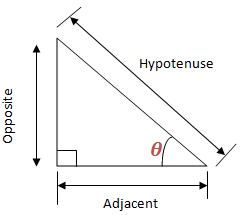The sides are given names according to their properties relating to the Angle .

Opposite side is opposite the Angle

Hypotenuse side is the longest side

Sine, Cosine and Tangent of an Angle using a Right Angled Triangle
Define sine, cosine and tangent of an angle using a right angled triangle

Trigonometry is good at finding the missing side or Angle of a right angled triangle.

The special functions, sine, cosine and tangent help us. They are simply one of a triangle divide by another. See similar triangles
below: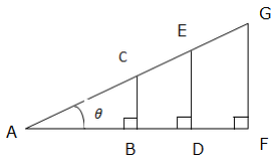The ratios of the corresponding sides are: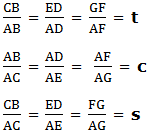Where by tc and s are constant ratios called tangent (t), cosine (c) and sine (s) of Angle respectively.

The right-angled triangle can be used to define trigonometrical ratios as follows:The short form of Tangent is tan, that of sine is sin and that of Cosine is cos.

The simple way to remember the definition of sine, cosine and tangent is the word SOHCAHTOA.

This means sine is Opposite (O) over Hypotenuse (H); cosine is Adjacent (A) over Hypotenuse (H); and tangent is Opposite (O) over Adjacent (A). Or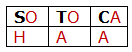Example 1

Given a triangle below, find sine, cosine and Tangent of an angle indicated.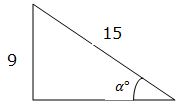Solution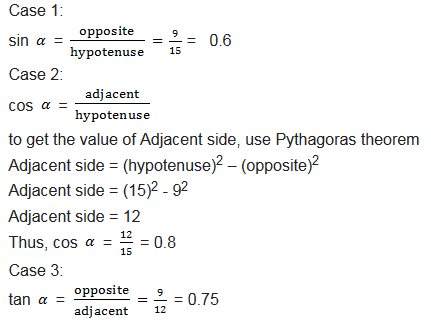Example 2
Given that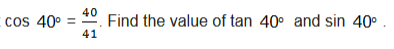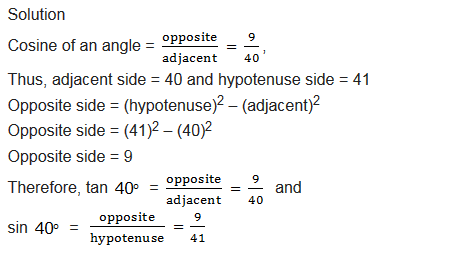#### Angles of Elevation and Depression

Angles of Elevation and Angles of Depression
Demonstrate angles of elevation and angles of depression

Angle of Elevation of an Object as seen by an Observer is the angle between the horizontal and the line from the Object to the Observer’s aye (the line of sight).

See the figure below for better understanding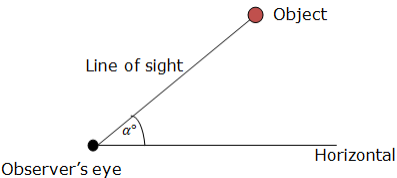The angle of Elevation of the Object from the Observer is α.

The angle of depression of the Object from the Object is β

Problems involving Angles of Elevation and Angles of Depression

Solve Problems involving angles of elevation and angles of depression

Example 5

From the top of a vertical cliff 40 m high, the angle of a depression of an object that is level with the base of the cliff is35. How far is the Object from the base of the cliff?
Solution

Angle of depression = 35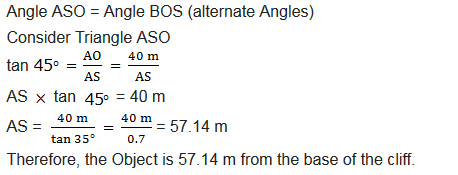#### Exercise 1

1. Use trigonometric tables to find the following:
1. cos 38.25
2. sin 56.5
3. tan 75
2. Use trigonometrical tables to find the value of x in the following problems.
1. sin x = 0.9107
2. tan x = 0.4621

3. Find the height of the tower if it casts a shadow of 30 m long when the angle of elevation of the sun is38.

4. The Angle of elevation of the top of a tree of one point from east of it and 56 m away from its base is25. From another point on west of the tree the Angle of elevation of the top is50. Find the distance of the latter point from the base of the tree.

5. A ladder of a length 15m leans against a wall and make an angle of30with a wall. How far up the wall does it reach?

2.Martin